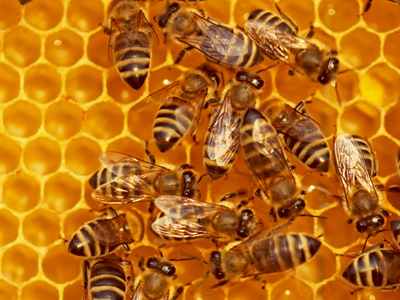A beehive increases by 7% a day.

# Level 7-8 Numbers - Percentages - Increases and Decreases

There are three ways to represent fractions of numbers - fractions, decimals and percentages. Percentages are a part of everyday life and you'll need to be able to calculate increase and decreases in percentages if you ever want to understand interest rates or pay rises!

To calculate a percentage increase, first divide the percentage by 100 and then multiply by the original figure. So, to find a 5% increase on £800, first divide 5 by 100 (0.05) and then multiply by 800 to get 40. Add this to the original figure to get £840.

To calculate a percentage decrease, follow the same steps but this time subtract 40 from 800 to give an answer of £760.

Put your numbers hat on and practise with the following quiz increases and decreases in percentages. If you can get the full 100% in this quiz, you've cracked it!

1.
A beehive increases by 7% a day. Initially there are 1,250 bees. How many will there be after a week?
1,620
1,800
2,000
2,007
A calculator is helpful for this kind of calculation! This problem is an example of compound increase. Problems like this can be solved using the compound interest formula:

M = P( 1 + i )n

Here, M = the final number of bees; P = the initial number of bees; i = the percentage increase (if it is a decrease, it will be negative; n = the number of days (but it could be weeks/months/years or any time period, depending on the problem) and it is the POWER to which (1 + i) has to be raised. Plug in the numbers and do the calculation
2.
Barbara bought a box of 35 pens for £40.25. She sold all the pens for £1.70 each. What was her percentage profit?
39.4%
40.7%
45.8%
47.8%
3.
Due to severe weather, a polar bear colony goes from 328 bears to 246. What percentage decrease is this?
20%
22%
25%
26%
Remember, the change is always compared with the original value
4.
Michelle bought a caravan for £13,500 and sold it two years later for £11,200. What is the percentage loss?
13%
14%
15%
17%
13,500 - 11,200 = 2,300
(13,500 ÷ 2,300) x 100 = 17.037....
5.
What percentage of 68 is 10.2?
15
17
17.5
20
68 ÷ 100 = 0.68
10.2 ÷ 0.68 = 14.70588235294118....
6.
A burger now costs £3.75 after a 3% reduction in price. What was the original price of the burger?
£2.88
£3.17
£3.87
£4.44
3.75 ÷ 97 = 0.0387
0.0387 x 100 = 3.87
7.
Pete's hourly wage goes up from £7.20 to £7.56. What percentage increase is this?
3%
4%
5%
6%
The increase is 36p. To work out the percentage 0.36 / 7.2 = 0.05 = 5%
8.
The price of a concert ticket is £33 after a 7% increase. What is the original price of the ticket?
£30.84
£31.11
£33.65
£34.86
33 ÷ 107 = 0.3084
0.3084 x 100 = 30.84
9.
The Green family bought a house five years ago for £97,000. They sell it for £185,000. What is their percentage profit (to the nearest 1%)?
81%
85%
89%
91%
185,000 - 97,000 = 88,000
97,000 ÷ 100 = 970
88,000 ÷ 970 = 90.722
10.
What percentage is 8.5 of 68?
12
12.5
13
13.5
8.5 ÷ 0.68 = 12.5